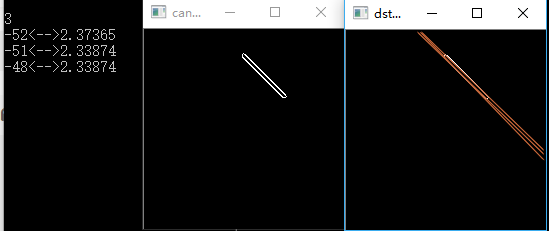2017-12-16 11:29:52 sinat_39805237 阅读数 2220

1局部处理2区域处理3使用霍夫变换的全局处理(一块不少，五块更好）

红包还不收？2011-11-18 20:54:01 liujia2100 阅读数 13484

Hough变换：

for(i=0;i<img.height;i++) //转化成灰度

{

for(j=0,n=0;n<img.width*3,j<img.width;n+=3,j++)

{ //gray 变量存储rgb转灰度的数据

gray= ((float)(img.image[lineBytes*i+n+2])+(float)(*(img.image+lineBytes*i+n+1))+(float)(*(img.image+lineBytes*i+n)))/3; //lineBytes 原图每行实际字节数

grayPic[i*img.width+j]=(byte)gray;//转换成的灰度图像放在grayPic中

}

}

int logNum; //边缘检测

memset(lpDIBBits,(byte)0,sizeof(byte)*img.height*img.width);

for(i=3;i<img.height-2;i++)

for(j=3;j<img.width-2;j++)

{

//logNum 变量 记录每次运算的值

logNum=16*grayPic[i*img.width+j]-grayPic[(i-2)*img.width+j]-grayPic[(i-1)*img.width+j-1]-2*grayPic[(i-1)*img.width+j]-grayPic[(i-1)*img.width+j+1]-grayPic[i*img.width+j-2]-2*grayPic[i*img.width+j-1]-2*grayPic[i*img.width+j+1]-grayPic[i*img.width+j+2]-grayPic[(i+1)*img.width+j-1]-2*grayPic[(i+1)*img.width+j]-grayPic[(i+1)*img.width+j+1]-grayPic[(i+2)*img.width+j];//log算子

if(logNum > 0)

lpDIBBits[i*img.width+j]=255;//边缘检测后的数据存放在lpDIBBits中

else

lpDIBBits[i*img.width+j]=0;

}

for(i=1;i<img.height;i++) //img.height原图高度

for(j=1;j<img.width;j++) //img.width 原图宽度

{

if(lpDIBBits[i*img.width+j]==255) //对边缘检测后的数据（存在lpDIBBits中）进行hough变化

{

for(k=1;k<ma;k++) //ma=180

{

p=(int)(i*cos(pi*k/180)+j*sin(pi*k/180));//p hough变换中距离参数

p=(int)(p/2+mp/2); //p值优化防止为负

npp[k][p]=npp[k][p]++; //npp对变换域中对应重复出现的点累加

}

}

}

kmax=0; //最长直线的角度

pmax=0; //最长直线的距离

n=0; //这一部分为寻找最长直线

for(i=1;i<ma;i++) //ma=180

for(j=1;j<mp;j++) //mp为原图对角线距离

{

if(npp[i][j]>yuzhi) //找出最长直线 yuzhi为中间变量用于比较

{

yuzhi=npp[i][j];

kmax=i; //记录最长直线的角度

pmax=j; //记录最长直线的距离

}

}

memset(temp,(byte)255,sizeof(byte)*img.width*img.height);//原图中坐标符合kmax和pmax的值

for(i=1;i<img.height;i++) //的集合即是最长的直线

for(j=1;j<img.width;j++)

{

if(lpDIBBits[i*img.width+j]==255)

{

p=(int)(i*cos(pi*kmax/180)+j*sin(pi*kmax/180));//pi=3.1415926

p=(int)(p/2+mp/2); //mp为原图对角线距离

if(p==pmax)

*(temp+i*img.width+j)=0; //存储图像数据 放在temp数组中

}

}2016-11-07 08:49:52 tina_ttl 阅读数 19640

# 1.直角坐标系与极坐标系中的直线表示

## 1.1 直角坐标系与极坐标系 中的点的表示及它们之间相互关系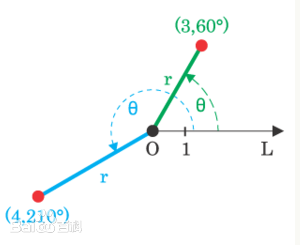• 1.在直角坐标系下，点P的坐标表示为(x,y)$(x,y)$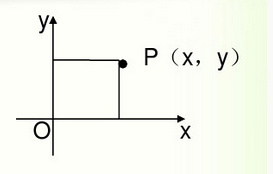• 2.在极坐标系下，点的坐标表示为(ρ,theta)$(\rho,theta)$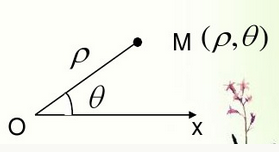• 3.某一点由极坐标转换为直角坐标

x=ρcosθ

y=ρsinθ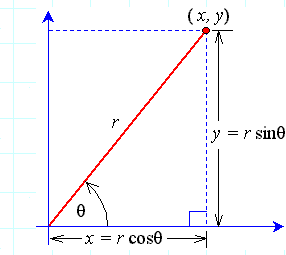注：图中的r与上面的ρ$\rho$是同样的描述

• 4.某一点直角坐标转换为极坐标

• θ$\theta$的具体取值还需要根据点P具体位于哪个象限进行调整，根据点的直角坐标可以判断其位于哪个象限，具体判断方法如下图如示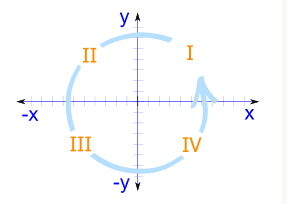• 根据点具体落到四个象限中的哪一个，在arctan结果 的基础上进行修正，arctan结果位于(-π/2,π/2)区间，具体方法如下：
• 点位于第一象限，则该点的极角为arctan
• 点位于第二象限，则该点的极角为arctan+π
• 点位于第三象限，则该点的极角为arctan+π
• 点位于第四象限，则该点的极角为arctan+2## 1.2 直角坐标系与极坐标系中的直线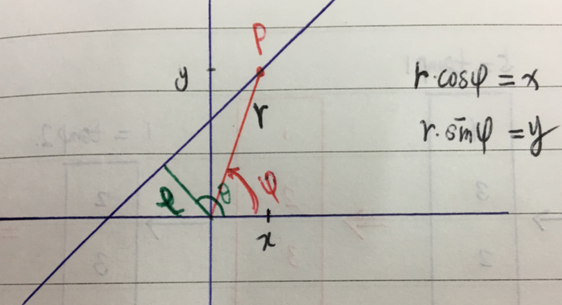• 首先，利用直线距离原点的距离的计算公式，可得下式
ρ=rcos(θφ)
• 然后，对该式利用三角函数展开，可以得到
ρ=rcos(θφ)=rcos(θ)cos(φ)+rsin(θ)sin(φ)
• 又根据点P的直角坐标系(x,y)与极坐标 (φ,r)$( \varphi,r )$之间 的关系rcos(φ)=x$r * cos(\varphi) = x$rsin(φ)=y$r * sin(\varphi) = y$，可以得到
ρ=xcosθ+ysinθ

# 2.利用Hough变换检测直线

## 2.1 线到点的Hough变换

### 2.1.1 通俗解释

• 给定一幅图像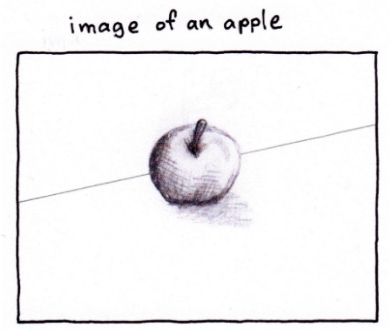• 检测边缘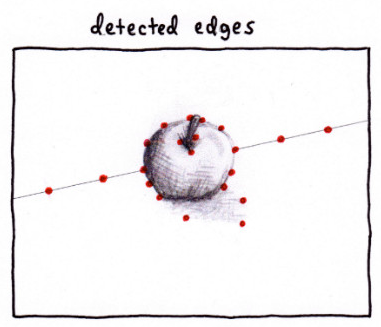• 对于任何一个边缘点，找到所有可能经过该点的直线，这些直线每一条都对应着参数空间中的一个点，而无穷多条之间对应于极坐标系下的点将形成一条极坐标系下的曲线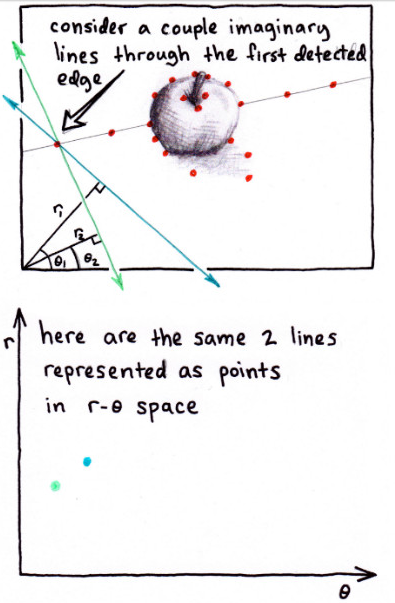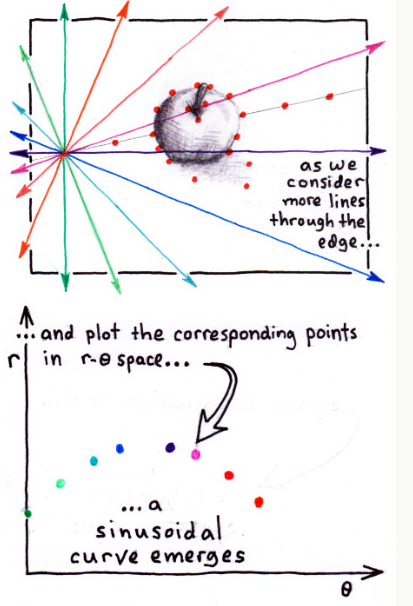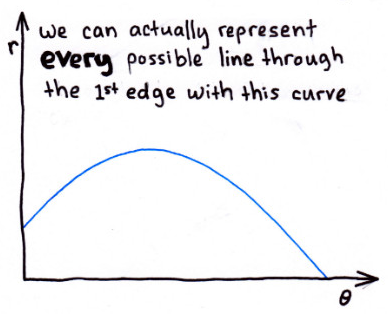• 重复上面过程，每个边缘点都对应于极坐标系下的一条曲线，那么，极坐标系下 （参数空间）所有这些曲线的交点一定是原图像中所有边缘点共同存在的直线！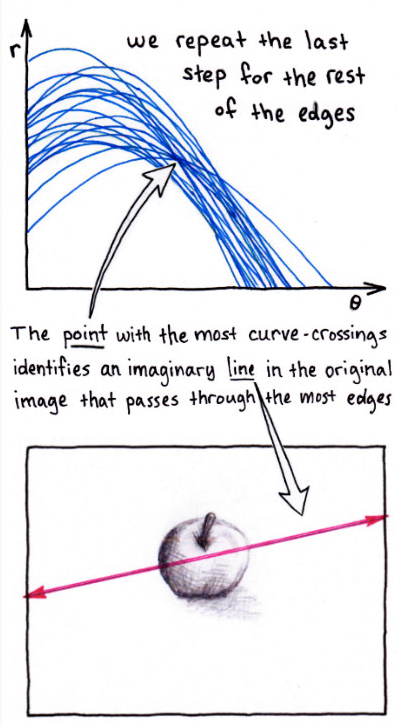## 2.2 Hough变换检测直线的实现

### 2.2.1 赵小川教程中的例子

• 假设一副图像大小为M×M$M\times M$，根据该图像尺寸，设定hough变换的参数空间取值范围
• 原点距离直线的距离：ρ[2N,2N]$\rho \in [-\sqrt{2}N,\sqrt{2}N]$
• 原点到直线的垂线与x轴正方向的夹角：θ[0,π]$\theta \in {[0,\pi] }$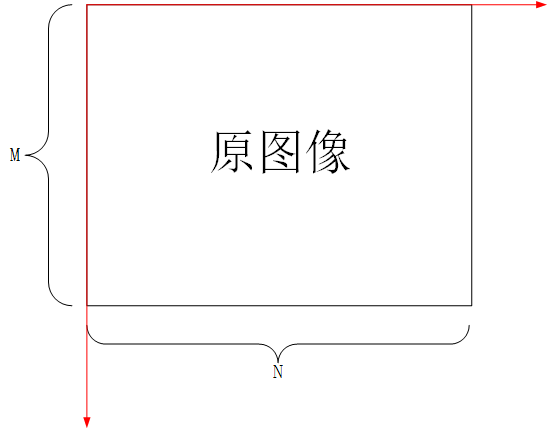• 按照参数的取值范围，将参数分为m×n$m \times n$个网格，即将θ[0,π]$\theta \in {[0,\pi] }$分为m份，将ρ[2N,2N]$\rho \in [-\sqrt{2}N,\sqrt{2}N]$分为n份，然后，设定一个m×n$m \times n$的累加单元，用来存储图像中某一条直线出现的次数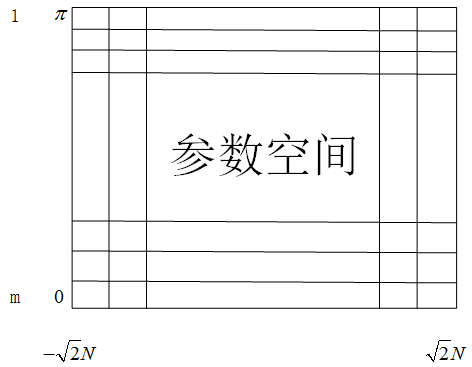• 接下来，对图像中原图像中每一个像素点(x,y)，分别进行如下操作：

• 在参数θ$\theta$对应的每一取值，分别按照公式ρ=xcosθ+ysinθ$\rho = xcos\theta +ysin\theta$计算相应的参数ρ$\rho$的取值
• 然后，在相应的参数累加单元中加1
• 按照上面操作，得到了一个累加单元，统计每个累加单元的取值，大于某个事先设定好的阈值，就认为该组参数便是图像空间内的直线的参数

### 2.2.2 matlab自带hough变换相关函数

matlab自带了Hough变换的相关函数：

• hough：实现霍夫变换，得到霍夫变换矩阵，用法如下
• [H, theta, rho] = hough(BW)
• 输入BW为二值边缘图像
• 输出H为参数空间参数的累加矩阵；
• 输出theta为数值向量，存储划分参数空间的参数θ$\theta$的所有取值；
• 输出rho为数值向量，存储划分参数空间的参数ρ$\rho$的所有取值
• [H, theta, rho] = hough(BW, ParameterName,ParameterValue)
• 这里增加了一组用来指定参数θ$\theta$ρ$\rho$的区间范围(及间隔)
• ‘RhoResolution’：指定参数θ$\theta$的区间的间隔
• ‘ThetaResolution’：指定参数ρ$\rho$的区间的间隔
• ‘Rho’：指定参数ρ$\rho$的区间的范围(及间隔)，例如：1:0.5:100（注：默认值为(0,norm(size(BW)))，即最大值为图像对角线长度，间隔为1）
• ‘Theta’：指定参数θ$\theta$的区间的范围(及间隔)，例如：0:0.5:50，（注：默认值为[-90°，90°)，间隔为1，单位为度）
• houghpeaks：在霍夫变换矩阵里找极值点

• peaks = houghpeaks(H, numpeaks)：找到hough变换矩阵中最大的numpeaks个值
• peaks = houghpeaks(…, param1, val1,param2, val2)：对最大值提取进行一些设置
• ’Threshold’：大于该阈值的点才会考虑是否为峰值，默认值为0.5max(H(:))$0.5*max(H(:))$
• ‘NHoodSize’：非极大值抑制窗的大小，默认为size(H)/50$size(H)/50$
• houghlines：从霍夫变换矩阵中提取线段

• lines = houghlines(BW, theta, rho,peaks)
• BW为原始图像边缘
• theta为hough返回的参数θ$\theta$的区间间隔
• rho为hough返回的参数ρ$\rho$的区间间隔
• peaks为houghpeaks提取出来的hough变换的极值点
• lines = houghlines(…, param1, val1,param2, val2)
• 输入值：’FillGap’：指定了与相同的霍夫变换相关的两条线段的距离。当两条线段之间的距离小于指定的值时，函数houghlines把线段合并为一条线段(默认的距离是20个像素)
• 输入值：’MinLength’：指定合并的线是保留还是丢弃。如果合并的线比val2指定的值短，就丢弃(默认值是40)
• 输出值：lines结构元胞，分别存放合并后的所有直线的相关信息
• point1：两元素向量[r1, c1]，指定了线段起点的行列坐标。
• point2：两元素向量[r2, c2]，指定了线段终点的行列坐标。
• theta：与线相关的霍夫变换的以度计量的角度。
• rho：与线相关的霍夫变换的ρ轴位置。

1. 读取图像并得到边缘图像

% 读取matlab自带的图像gantrycrane，并提取边缘
I  = rgb2gray(RGB);
BW = edge(I,'canny'); %提取边缘
subplot(1,2,1);imshow(RGB);title('原始图像');
subplot(1,2,2);imshow(BW);title('边缘图像');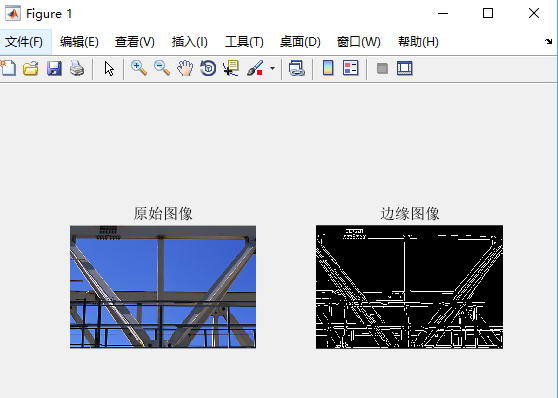2. 利用Hough函数对输入图像边缘进行hough变换

% 对原始图像边缘进行hough变换，指定参数的分别率（取样间隔），区间利用默认值
[H,T,R] = hough(BW,'RhoResolution',0.5,'ThetaResolution',0.5);
• H为hough transform矩阵，每个元素分别存放的相应参数对应的累加
• T为存放theta的采样值的向量
• R为存放rho采样值的向量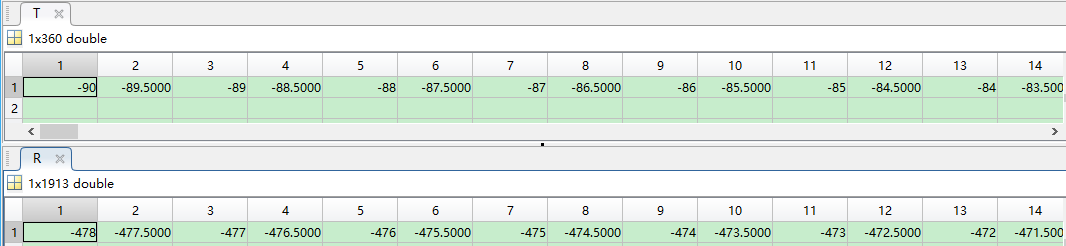3.显示hough变换的结果，即显示矩阵H（即bins）

% 显示hough变换的结果
figure;
'InitialMagnification','fit');
title('Limited Theta Range Hough Transform of Gantrycrane Image');
xlabel('\theta'), ylabel('\rho');
axis on, axis normal;
colormap(hot)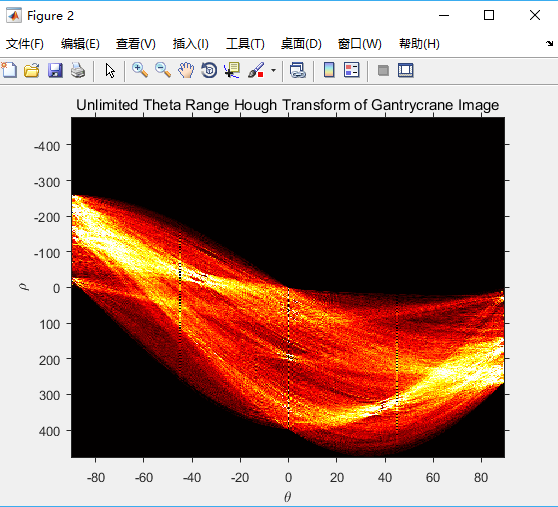[H,T,R] = hough(BW, 'Theta', 44:0.5:46);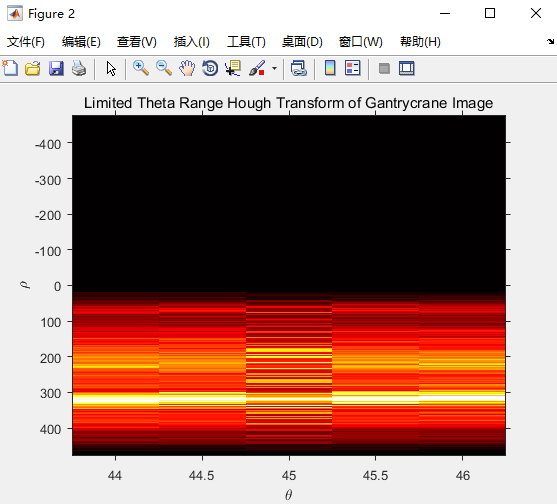4.显示hough变换结果中的极值点

% 显示霍夫变换矩阵中的极值点
P = houghpeaks(H,10,'threshold',ceil(0.3*max(H(:)))); % 从霍夫变换矩阵H中提取50个极值点
x = T(P(:,2));%极值点的theta值，即P的第二列存放的是极值点的theta值
y = R(P(:,1));%极值点的rho值，即P的第二列存放的是极值点的rho值
hold on;plot(x,y,'s','color','black');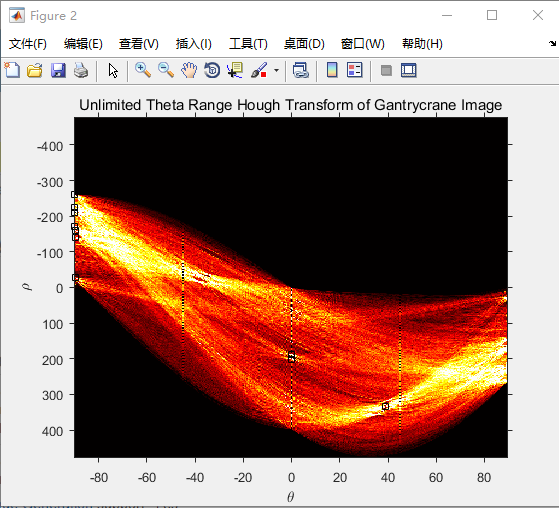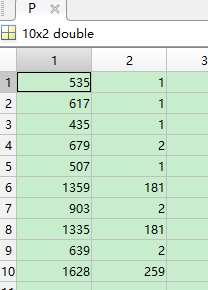5.将提取得到的极值点变换回原图像，得到提取的直线

% 找原图中的直线
lines = houghlines(BW,T,R,P,'FillGap',18,'MinLength',80);

• ‘FillGap’：当两条直线之间距离小于该阈值时，两条直线被合并为一条直线
• ‘MinLength’：保留的直线的最短长度（即小于该阈值的直线将被删除）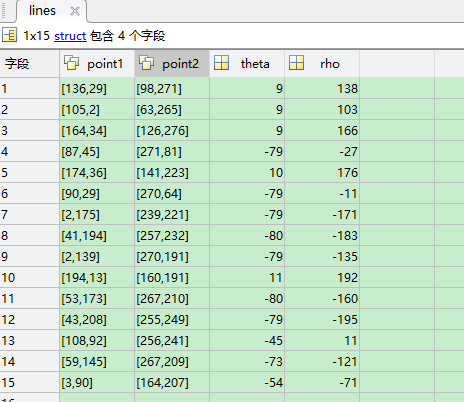6.在原图像中绘制得到的直线

% 绘制提取得到的直线
figure, imshow(I), hold on
max_len = 0;
for k = 1:length(lines)
% 绘制第k条直线
xy = [lines(k).point1; lines(k).point2];
plot(xy(:,1),xy(:,2),'LineWidth',2,'Color','green');

% 绘制第k条直线的起点（黄色）、终点（红色）
plot(xy(1,1),xy(1,2),'x','LineWidth',2,'Color','yellow');
plot(xy(2,1),xy(2,2),'x','LineWidth',2,'Color','red');

% 计算第k条直线的长度，保留最长直线的端点
len = norm(lines(k).point1 - lines(k).point2);
if ( len > max_len)
max_len = len;
xy_long = xy;
end
end
title('提取到的直线');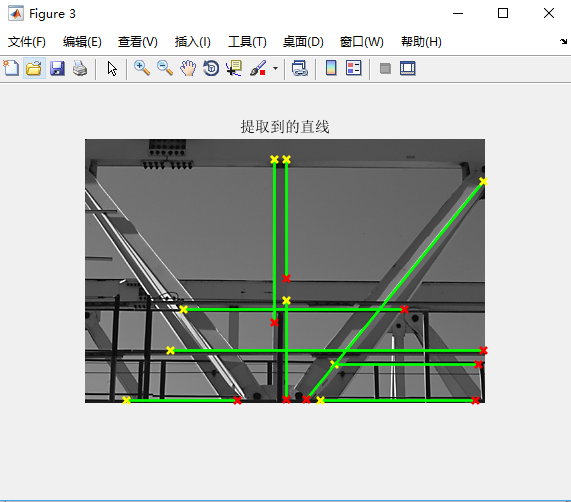% 以红色线高亮显示最长的线
plot(xy_long(:,1),xy_long(:,2),'LineWidth',2,'Color','red');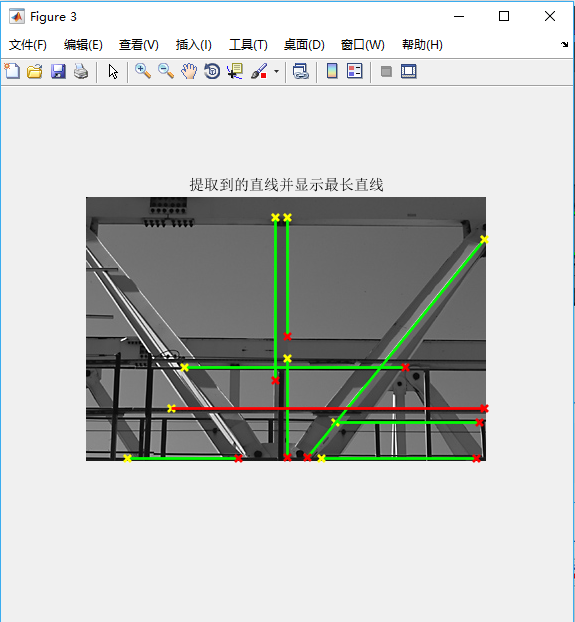# 3.Hough变换的优缺点

• 抗噪能力强
• 对边缘间断不敏感

• 运算量大：对图像中每一个像素点，都需要计算所有的theta对一个的rho
• 占用内存多

Hough变换利用的是一种投票思想

Reference

1. stackoverflow=explain hough transformation．http://stackoverflow.com/questions/4709725/explain-hough-transformation
2. Polar and Cartesian Coordinates． https://www.mathsisfun.com/polar-cartesian-coordinates.html
4. http://www.nabla.hr/Z_MemoHU-015.htm
5. hough变换是如何检测出直线和圆的？ http://blog.163.com/yuyang_tech/blog/static/21605008320130233343990/
6. 现代数字图像处理技术提高及应用案例详解（MATLAB版）. 赵小川

2018-12-05 20:10:03 yql_617540298 阅读数 85

# 一、霍夫变换思想(Hough Transform)

霍夫变换主要是利用图片所在的空间和霍夫空间之间的变换，将图片所在的直角坐标系中具有形状的曲线或直线映射到霍夫空间的一个点上形成峰值，从而将检测任意形状的问题转化成了计算峰值的问题；

即在图片所在的直角坐标系的一个直线，转换到霍夫空间便成了一点，并且是由多条直线相交而成，我们统计的峰值也就是该相交点的橡胶线的条数。

Hough变换的基本原理在于利用点与线的对偶性，将原始图像空间的给定曲线通过曲线表达形式变为参数空间的一个点。这样就把原始图像给定曲线的检测问题，转化为检测参数空间的峰值问题，也就是把检测整体特性转化为检测局部特性。比如直线，椭圆，圆，弧线等。

（1）设已知一黑白图像上画了一条直线，要求出这条直线所在的位置。我们知道，直线的方程可以用y=k*x+b 来表示，其中k和b是参数，分别是斜率和截距。也就是说，我们将原始图像需要检测的直线，表示成y = k*x + b, 只要找出唯一的k，b即可检测出该直线。该直线在原始图中是一系列离散点的集合，过该直线上某一点(x0,y0)的所有直线的参数都会满足方程y0=kx0+b。即点(x0,y0)确定了一条直线。而方程y0=kx0+b在参数k--b平面上是一条直线，(也可以是方程b=-x0*k+y0对应的直线).即点（x0,y0）在参数空间确定了一条直线。

（2）这样，图像x--y平面上的一个前景像素点就对应到参数平面上的一条直线。因此，图像x-y内需检测直线上的N个点，在参数平面会有N条直线。而图像x-y内的直线有唯一一个k，b，因此，相应的参数平面N条直线必然有唯一一个交点。

（3）图示：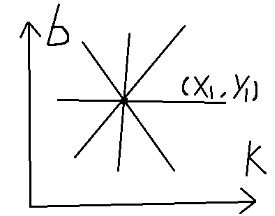# 二、倾斜文本校正

import os
import cv2
import math
import numpy as np
from scipy import misc, ndimage
from PIL import Image

count = os.listdir(filepath)

#for filename in os.listdir(filepath):
for j in range(1,len(count)+1):
img1 = Image.open(filepath+'/rgb_'+str(j).zfill(4)+'.png')
img1 = img1.convert('RGBA')
#print("img",img)
#img1 = Image.open(filepath+'/'+str(j).zfill(4)+'.png')
n = img.shape
m = img.shape
#print(m,n)
gray = cv2.cvtColor(img,cv2.COLOR_BGR2GRAY)
edges = cv2.Canny(gray,50,150,apertureSize = 3)

#霍夫变换
lines = cv2.HoughLines(edges,1,np.pi/180,0)
for rho,theta in lines:
a = np.cos(theta)
b = np.sin(theta)
x0 = a*rho
y0 = b*rho
x1 = int(x0 + 1000*(-b))
y1 = int(y0 + 1000*(a))
x2 = int(x0 - 1000*(-b))
y2 = int(y0 - 1000*(a))
if x1 == x2 or y1 == y2:
continue
t = float(y2-y1)/(x2-x1)
rotate_angle = math.degrees(math.atan(t))
if rotate_angle > 45:
rotate_angle = -90 + rotate_angle
elif rotate_angle < -45:
rotate_angle = 90 + rotate_angle
rotate_img = ndimage.rotate(img, rotate_angle)
misc.imsave(resultpath+'/'+'rgb_'+str(j).zfill(4)+'.png', rotate_img)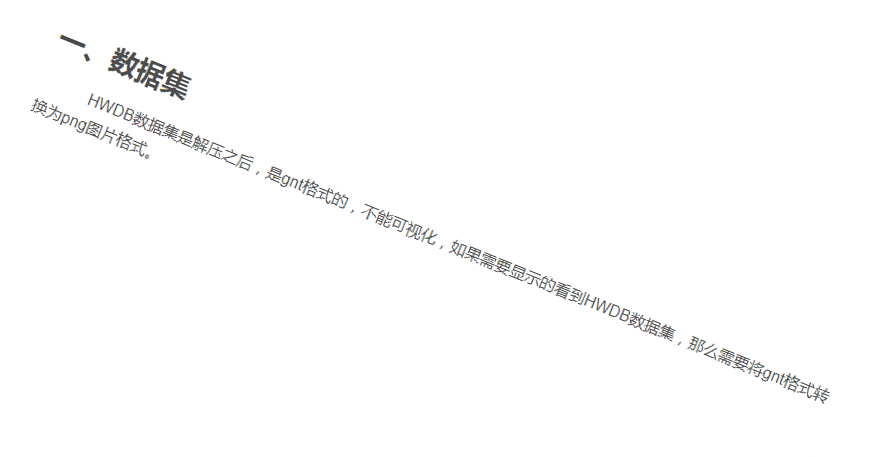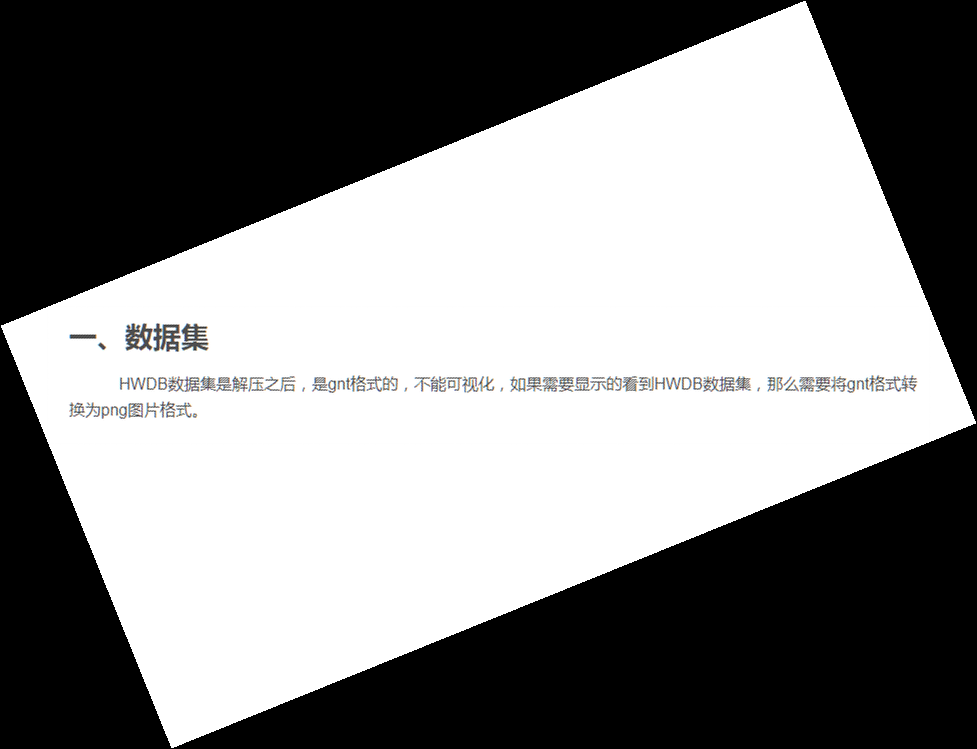region = img1.crop((5,5,10,10))
region = np.array(region)
R = np.mean(region[:,:,0])
G = np.mean(region[:,:,1])
B = np.mean(region[:,:,2])

card = Image.new("RGBA", (m, n), (int(R),int(G),int(B)))
card.paste(rotate_image, (0, 0, m, n), rotate_image)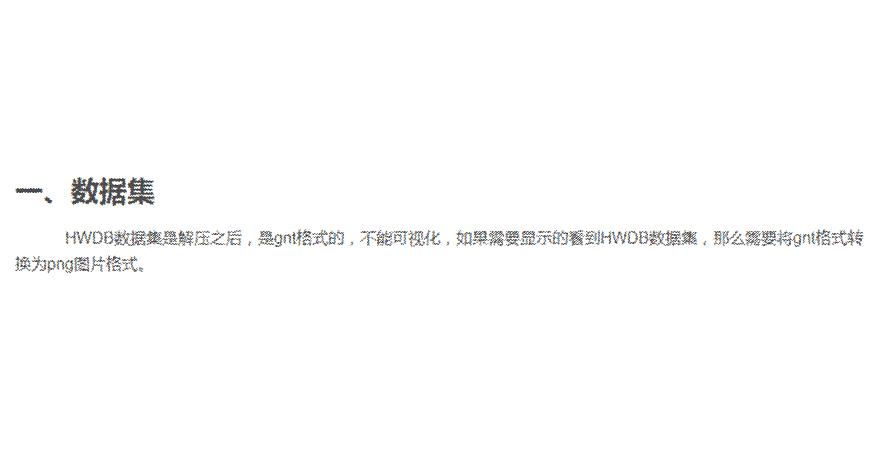2018-03-23 15:33:22 Mirror33 阅读数 284

# 霍夫线检测原理：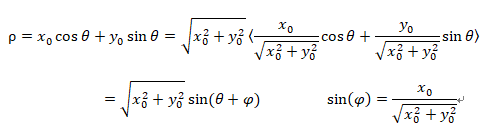ρ，θ就是一对hough空间的变量表示。若将ρ，θ看成直角坐标空间,一个点(x0, y0)就是一个关于ρ，θ的正弦曲线。同样，直线上的其他点（Xn,Yn）也会构成一组关于ρ，θ的正弦曲线，这样势必存在一个关于ρ，θ相交（即垂直点（r,θ））。于是乎， 一条直线能够通过在极坐标下寻找交于一点的曲线数量来检测，如果越多曲线交于一点，就意味着这个交点表示的直线由更多的点组成。我们可以通过设置直线上点的阈值来定义多少条曲线交于一点我们才认为检测到了一条直线。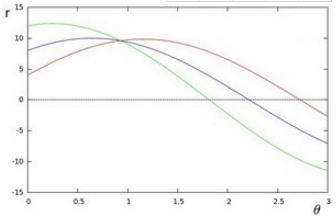template<typename T>
int myRound(T value)
{
return (int)(value + (value >= 0 ? 0.5 : -0.5));
}
/*

src-->输入的二值图像   lines-->输出的线的坐标(r,θ)
rho-->极坐标r的步长    theta-->极坐标θ的步长
threshold-->交点个数阈值 linesMax-->线条数的最大值

*/
void doHoughLinesStandard(Mat &src, vector<Vec2f>&lines,float rho, float theta, int threshold,int linesMax)
{
float irho = 1 / rho;
const int width = src.cols;
const int height = src.rows;
int i, j, n, r;
//将r,theta离散化,形成离散化的hough空间,类似一个mat矩阵/图像,用于统计角点的个数.
//角度的离散值个数(180),θ∈[0,180°].Δθ = 1.
int numangle = (int)(CV_PI / theta);
//numrho本来的范围是[-D,+D],这里放大(D=(w + h)).且有效范围从1开始,故扩展至[1,2D+1].Δr = 1.
int numrho = (int)(((width + height) * 2 + 1) / rho);//这里+1是因为要隔开极坐标空间的左右部分.
//极坐标空间的上下空留一行左右空留一列,为后面的4邻域比较做准备.
int **_accum = new int*[(numangle + 2)];
for (i = 0; i < (numangle + 2); i++) {
_accum[i] = new int[(numrho + 2)];
memset(_accum[i], 0x00, sizeof(int)*(numrho + 2));
}

vector<int> _sort_buf;
//正余弦参数表,以后通过查表的方式来获取值，一种加速手段,以空间换时间。
float* _tabSin = new float[numangle];
float* _tabCos = new float[numangle];
float ang = 0.0f;
for (n = 0; n<numangle; ang += theta, n++) {
_tabSin[n] = sin(((double)ang)*irho);
_tabCos[n] = cos(((double)ang)*irho);
}
//step1:fill accumulator
for (i = 0; i<src.rows; i++) {
for (j = 0; j<src.cols; j++) {
//将每个非零点转换为霍夫空间的离散正弦曲线，并统计。
if (src.data[i*((int)(src.step)) + j] != 0) {
for (n = 0; n<numangle; n++) {
r = myRound(j*_tabCos[n] + i*_tabSin[n]);
r += (numrho - 1) / 2;//r的范围[1,(2D+1)]决定这里需要+D
_accum[n + 1][r + 1]++;
}
}
}
}
//stp2:find local maximums
/*霍夫空间，局部最大点，采用四邻域判断、比较，如果不判断局部最大值，同时选用次大值和最大值，
可能会是两个相邻的直线，但实际上就是一条直线。选用最大值，去除离散的近似计算带来的误差，合并近似曲线。
*/
for (r = 0; r<numrho; r++) {
for (n = 0; n<numangle; n++) {
if (_accum[(n + 1)][r + 1] > threshold &&
_accum[(n + 1)][r + 1] > _accum[(n + 1)][r] && _accum[(n + 1)][r + 1] >= _accum[(n + 1)][r + 2] &&
_accum[(n + 1)][r + 1] > _accum[(n)][r + 1] && _accum[(n + 1)][r + 1] >= _accum[(n + 2)][r + 1]) {
_sort_buf.push_back(n + 1); _sort_buf.push_back(r + 1);
}
}
}
//依据霍夫空间分辨率，计算直线的实际r，theta参数
for (i = 0; i<_sort_buf.size() && i<linesMax; i += 2) {
int nx = _sort_buf[i]-1;//得到θ的坐标,单位为度.-1的原因是刚开始平移了1个单位，下同.
int rx = _sort_buf[i + 1]-1;
float rho_res = (rx - (numrho - 1)*0.5f)*rho;//r恢复原来的值须-D.
float angle_res =  nx*theta;//将度转化为弧度
lines.push_back(Vec2f(rho_res, angle_res));
}

if (_accum) {
for (i = 0; i < numangle; i++) {
if (_accum[i]) {
delete[]_accum[i]; _accum[i] = NULL;
}
}
delete[] _accum; _accum = NULL;
}
if (_tabSin) {delete[] _tabSin; _tabSin= NULL;}
if (_tabCos) { delete[] _tabCos; _tabCos = NULL; }
}

void xxHoughTransform()
{
Mat midImage, dstImage;
Canny(src, midImage, 50, 180);
cvtColor(midImage, dstImage, CV_GRAY2BGR);
vector<Vec2f> lines;
doHoughLinesStandard(midImage, lines, 1.0, CV_PI / 180,52, 100);
//HoughLines(midImage, lines, 1, CV_PI / 180,50);
cout << lines.size() << endl;
for (size_t i = 0; i < lines.size(); i++)
{
float rho = lines[i], theta = lines[i];
cout << rho << "<-->" << theta << endl;
Point pt1, pt2;
double a = cos(theta), b = sin(theta);
double x0 = a*rho, y0 = b*rho;
pt1.x = cvRound(x0 + 1000 * (-b));
pt1.y = cvRound(y0 + 1000 * (a));
pt2.x = cvRound(x0 - 1000 * (-b));
pt2.y = cvRound(y0 - 1000 * (a));
line(dstImage, pt1, pt2, Scalar(55, 100, 195), 1, CV_AA);
}
imshow("src000", src);
imshow("canny000", midImage);
imshow("dst000", dstImage);
waitKey();
}